How Cheenta works to ensure student success?
Explore the Back-Story

# AMC 8 2019 Problem 20 | Fundamental Theorem of AlgebraTry out this beautiful algebra problem number 2 from AMC 8 2019 based on the Fundamental Theorem of Algebra.

## AMC 8 2019 Problem 20:

How many different real numbers" width="10" height="8"> satisfy the equation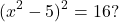" width="119" height="19">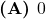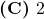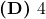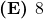### Key Concepts

Algebra

Value

Telescoping

AMC 8, 2019, Problem 20

## Try with Hints

The given equation is

and that means

Among both cases, if

then,

and that means we have 2 different real numbers that satisfy the equation.

and if we take another case, then

and so,

and that means we have 2 different real numbers in this case too that satisfy the equation. So total 2+2=4 real numbers that satisfy the equation.

Cheenta Numerates Program for AMC - AIME

### Subscribe to Cheenta at Youtube

Try out this beautiful algebra problem number 2 from AMC 8 2019 based on the Fundamental Theorem of Algebra.

## AMC 8 2019 Problem 20:

How many different real numbers" width="10" height="8"> satisfy the equation" width="119" height="19">### Key Concepts

Algebra

Value

Telescoping

AMC 8, 2019, Problem 20

## Try with Hints

The given equation is

and that means

Among both cases, if

then,

and that means we have 2 different real numbers that satisfy the equation.

and if we take another case, then

and so,

and that means we have 2 different real numbers in this case too that satisfy the equation. So total 2+2=4 real numbers that satisfy the equation.

Cheenta Numerates Program for AMC - AIME

### Subscribe to Cheenta at Youtube

This site uses Akismet to reduce spam. Learn how your comment data is processed.

### Knowledge Partner